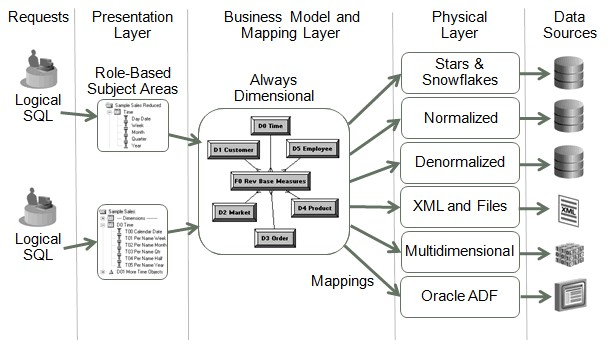# OBIEE - Running Sum (RSum)

Running Sum is a display function.

This function calculates a running sum based on records encountered so far.

The “By” statement resets its values for each group.

## Example

``````SELECT
QUARTER,
MONTH,
revenue,
RSUM(revenue)            AS RUNNING_SUM,
RSUM(revenue BY QUARTER) AS RUNNING_SUM_BY_QUARTER
FROM
sales_subject_area```
```

Result:

``````QUARTER   MONTH    REVENUE    RUNNING_SUM   RUNNING_SUM_BY_QUARTER
1         JAN      100.00     100.00        100.00
1         FEB      200.00     300.00        300.00
1         MAR      100.00     400.00        400.00
2         APRIL    100.00     500.00        100.00
2         MAY      300.00     800.00        400.00
2         JUNE     400.00     1200.00       800.00
3         JULY     500.00     1700.00       500.00
3         AUG      500.00     2200.00       1000.00
3         SEPT     500.00     2700.00       1500.00
4         OCT      300.00     3000.00       300.00
4         NOV      200.00     3200.00       500.00
4         DEC      100.00     3300.00       600.00
```
```

## How to

### Show a running sum in a graph

When your data have the same grain than the graph, you will get no problem. For instance, if you ask a week graph and that your data have only one line per week.

If your data have more than one line per week, you need to get the amount of the last line.

Unfortunately, OBIEE doesn't give the possibilitiy to set a Last aggregate rule for your column.

The following solution:

• calculates the first line per Week
• shows only the total amount by week of the first line
• and then calculates the Running Sum of the result.
``````RSUM
(
CASE
WHEN RCOUNT("Date"."Year Week" BY "Date"."Year Week") = 1 THEN
SUM("Fact"."Amount" BY "Date"."Year Week")
END
)```
```

Requisites:

• The column aggregation rule rule must then be set to Max or Min.
• The sort order of the analytics must be set on each column of the analytics that forms the unique key. Ie for this example: The Week and others columns that permits to select the rows uniquely.

## Documentation / Reference

Recommended PagesOBIEE - (Display Function|Derived Measures) (Rank, TopN, Median, )

Oracle BI Answers simplifies the use of derived measures i.e. measures that are computed on a query result set such as ranks, Ntiles, standard deviations, running totals, moving averages, and moving medians....OBIEE - Functions

The home page of OBIEE functions of the logical sql and from BI Server. See Logical SQL Reference